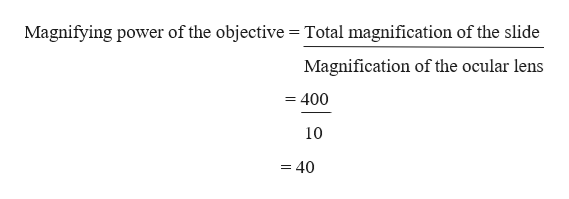# If the total magnification of a slide is 400x and the ocular lenses are 10x, what is the magnifying power of the objective being used?

Question
15 views

If the total magnification of a slide is 400x and the ocular lenses are 10x, what is the magnifying power of the objective being used?

check_circle

star
star
star
star
star
1 Rating
Step 1

Magnification is the process of enlarging the apparent size. This enlargement is quantified by a calculated number.

Step 2

In order to ascertain the total magnification when viewing an image with a compound microscope, the power of the objective lens is multiplied by the power of the eyepiece. Given total magnification of the sl...help_outlineImage TranscriptioncloseMagnifying power of the objective Total magnification of the slide Magnification of the ocular lens = 400 10 = 40 fullscreen

### Want to see the full answer?

See Solution

#### Want to see this answer and more?

Solutions are written by subject experts who are available 24/7. Questions are typically answered within 1 hour.*

See Solution
*Response times may vary by subject and question.
Tagged in

### Other